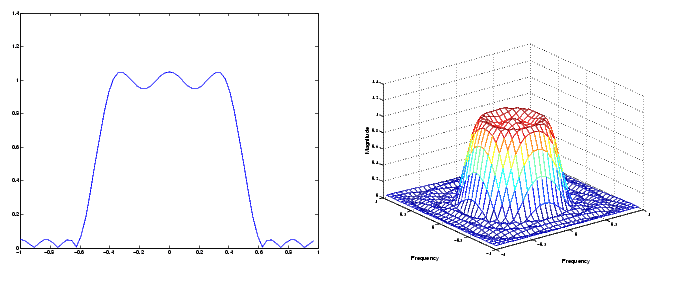Image Processing Toolbox User's GuideFrequency Transformation Method

The frequency transformation method transforms a one-dimensional FIR filter into a two-dimensional FIR filter. The frequency transformation method preserves most of the characteristics of the one-dimensional filter, particularly the transition bandwidth and ripple characteristics. This method uses a transformation matrix, a set of elements that defines the frequency transformation.

The toolbox function `ftrans2` implements the frequency transformation method. This function's default transformation matrix produces filters with nearly circular symmetry. By defining your own transformation matrix, you can obtain different symmetries. (See Jae S. Lim, Two-Dimensional Signal and Image Processing, 1990, for details.)

The frequency transformation method generally produces very good results, as it is easier to design a one-dimensional filter with particular characteristics than a corresponding two-dimensional filter. For instance, the next example designs an optimal equiripple one-dimensional FIR filter and uses it to create a two-dimensional filter with similar characteristics. The shape of the one-dimensional frequency response is clearly evident in the two-dimensional response.

• ```b = remez(10,[0 0.4 0.6 1],[1 1 0 0]);
h = ftrans2(b);
[H,w] = freqz(b,1,64,'whole');
colormap(jet(64))
plot(w/pi-1,fftshift(abs(H)))
figure, freqz2(h,[32 32])```

One-Dimensional Frequency Response (left) and Corresponding Two-Dimensional Frequency Response (right)FIR Filters Frequency Sampling Method